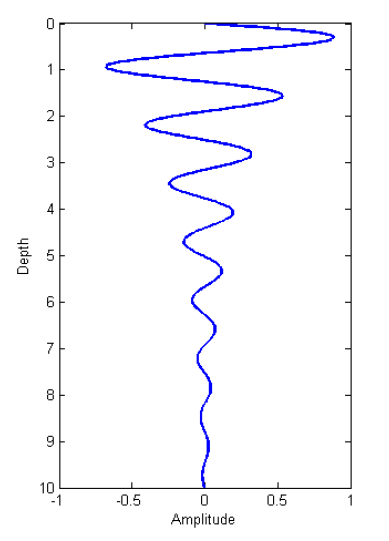# WavelengthFig. 46 A plane harmonic wave as it propagates into the earth.

Wavelength defines the physical distance a wave travels during a single oscillation. As it turns out, the wavelength for EM waves depends on the real component of the wavenumber ($$\alpha$$) and is given by:

$\lambda = \frac{2\pi}{\alpha} = \frac{2\pi}{\omega} \left ( \frac{\mu \epsilon}{2} \left [ \left ( 1 + \frac{\sigma^2}{\epsilon^2 \omega^2} \right )^{1/2} + 1 \right ] \right )^{-1/2}$

As we can see from the previous equation, higher frequencies correspond waves with to shorter wavelengths.

## EM Wavelengths for Various Materials

The table below shows wavelengths for EM waves travelling in certain rocks at various frequencies. This is meant to serve as a general guide, as rock types are classified by a range of physical properties values which can lead to order of magnitude differences in wavelength.

Type

$$\sigma$$

$$\mu_r$$

$$\epsilon_r$$

$$\lambda$$ (1Hz)

$$\lambda$$ (1kHz)

$$\lambda$$ (1MHz)

$$\lambda$$ (1GHz)

Air

0 S/m

1

1

299,800 km

299,800 m

299.8 m

0.2998 m

Sea Water

3.3 S/m

1

80

1.7 km

55 m

1.7 m

0.032 m

Igneous

$$10^{-4}$$ S/m

1

5

316 km

10,000 m

132 m

0.13 m

Sedimentary (dry)

$$10^{-3}$$ S/m

1

4

100 km

3,200 m

90 m

0.15 m

Sedimentary (wet)

$$10^{-2}$$ S/m

1

25

32 km

1,000 m

30 m

0.06 m

Sulphide Skarn

$$10^{2}$$ S/m

1

5

0.32 km

10 m

0.32 m

0.01 m

Magnetite Skarn

$$10^{2}$$ S/m

2

5

0.22 km

7 m

0.22 m

0.007 m

## Approximations

### Quasi-Static Approximation

In quasi-static regime ($$\epsilon\omega \ll \sigma$$), the wavelength simplifies to:

$\lambda = 2\pi\sqrt{ \frac{2}{\omega \mu \sigma} } = 2\pi\delta$

### Wave Regime Approximation

In the wave regime ( $$\epsilon \omega \gg \sigma$$ ), the wavelength simplifies to:

$\lambda = \frac{1}{\omega \sqrt{\mu \epsilon}}$